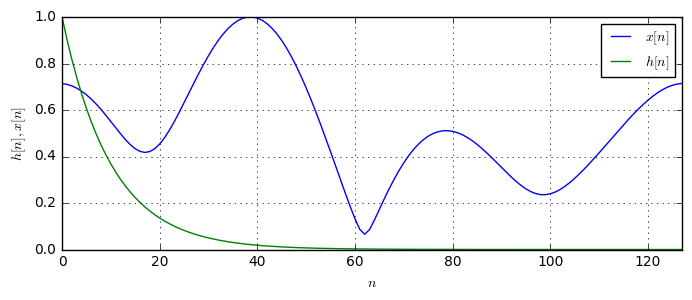# Circular Convolution¶

In a previous post, we have explained the importance of the convolution operation for signal processing and signal analysis. We have described the convolution integral and illustrated the involved functions.

In this post we will focus on an operation called Circular convolution which is strongly related to the conventional convolution (also called linear convolution) we have described before. Let us reconsider the normal, linear convolution in the discrete domain. Given two sequences $x[n]$ and $h[n]$, their convolution is given by

$$(x*h)[n] = \sum_{n'=-\infty}^{\infty}x[n']\cdot h[n-n'], \quad n=-\infty,\dots,\infty.$$

The linear convolution lets one one sequence slide over the other and sums the overlapping parts. The circular convolution of two sequences $x[n], h[n]$ is now considering a wrap-around of the sequences after a period of N samples. So, the circular convolution is defined by

$$(x\otimes h)[n]=\sum_{n'=0}^{N-1}x[n']\cdot h[(n'-n)_N], \quad n=0,\dots,N-1,$$

where $(n'-n)_N$ gives the remainder of $n'-n$ divided by $N$. For example, $(-1)_N=N-1$. This means, if the index for $x[(n'-n)_N]$ would leave the range $0,\dots,N-1$ to the left, it would wrap around and come in from the right again. This means that the circular convolution is periodic with length $N$.

In discrete domain, the convolution theorem actually holds only for the circular convolution: Let $X[k]$ and $H[k]$ be the N-point DFT of $x[n]$ and $h[n]$. Then the convolution theorem in discrete domain states

$$X[k]H[k]=\text{DFT}_N\{x[n]\otimes h[n]\}.$$

However, we can emulate a linear convolution by performing an appropriate zero-padding to both sequences and perform longer DFTs.

Let us now try to describe the circular convolution in pictures. First, we generate some random signal that we use to demonstrate the convolution.

N = 128   # the sequence length
# Generate some random sequence we use for the convolution
x = abs(N*np.fft.ifft(np.random.randn(5)+1j*np.random.randn(5), N))
# use some exponential sequence for the second function
h = np.exp(-0.1*np.arange(N))

plt.plot(x, label='$x[n]$');
plt.plot(h, label='$h[n]$');Let us now perform the circular convolution according to the formula of the convolution sum:

def showCircularConvolution(n0):
n = np.arange(N)
plt.subplot(211)
plt.plot(n, x[n], label='$x[n]$')
plt.plot(n, h[(n0-n)%N], label='$h[(n_0-n)_N]$')
plt.plot(n, x[n] * h[(n0-n)%N], label=r'$x[n]\cdot h[(n_0-n)_N]$')

plt.subplot(212)
hx = np.fft.ifft(np.fft.fft(x[n]) * np.fft.fft(h[n])) # use convolution theorem
plt.plot(n, abs(hx[n]))
current = sum(x[np] * h[(n0-np) % N] for np in n)     # direct calculation for the convolution
plt.plot(n0, current, 'ro')Run code interactively  Hide



As we see, the red area wraps around within the period. Furthermore, we have applied the convolution theorem to calculate the overall convolution result and confirmed the result with a direct calculation of the circular convolution.

# Conclusion¶

1. The circular convolution is defined on time-limited sequences of length $N$.
2. The circular convolution is periodic with period $N$.
3. In the circular convolution, the shifted sequence wraps around the summation window, when it would leave the region.
4. In the finite discrete domain, the convolution theorem holds for the circular convolution, not for the linear convolution. Linear convolution can be obtained by appropriate zero-padding of the sequences.Do you have questions or comments? Let's dicuss below!

### Related posts

DSPIllustrations.com is a participant in the Amazon Services LLC Associates Program, an affiliate advertising program designed to provide a means for sites to earn advertising fees by advertising and linking to amazon.com, amazon.de, amazon.co.uk, amazon.it.